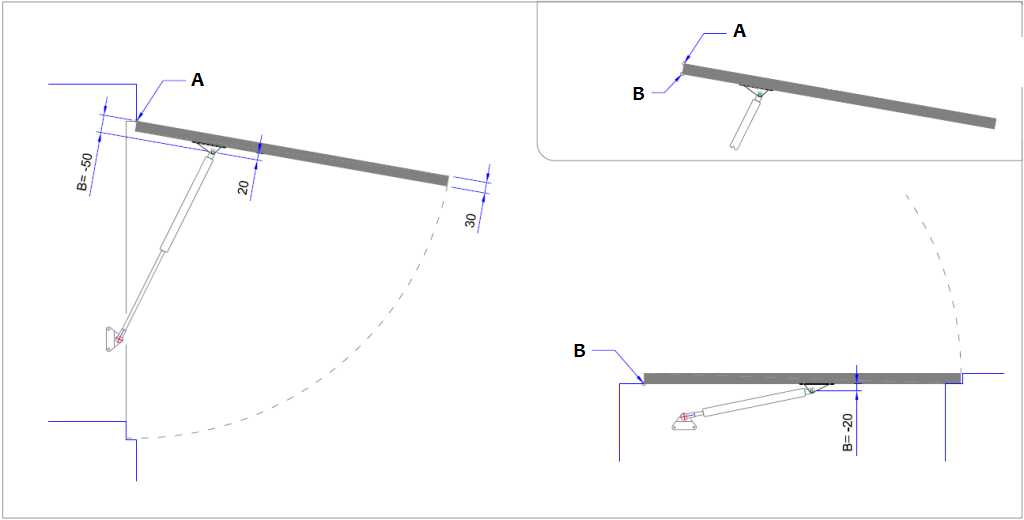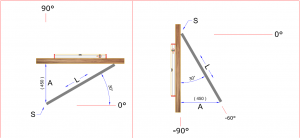## Why is the B measurement important? And how do I change that while maintaining the operation of the gas springs?

In the result of the Calculation tool the B measurement, along with the A measurement, displays the position of the centre of the end fitting of the gas spring to the cover.

Both of these distances will be shown from the centre of the pivot point from the cover.

The A measurement is a distance measured along the cover and the B measurement is a distance measured perpendicular to that.

The A measurement is a value that comes out of the gas spring calculation while the B measurement is directly dependent on the distance between the end fitting of the gas strut and the surface of the cover.

The Calculation tool sets the B measurement on the basis of the entered thickness of the cover and the position of the pivot point relative to the cover with thereby the point of attachment of the gas spring being 20 mm away from the cover surface as standard.

Below are some examples of the B measurement set by the Calculation tool (A = hinge on top, B = hinge on bottom):Once ‘Calculate’ is pressed in in Calculation tool (in Step 2), the B measurement is determined by a standard calculation rule and gas spring application is calculated.

The B measurement, which is then as shown in the result of the calculation, must correspond to the B distance from the point of attachment of the gas spring as it will eventually be mounted on the cover.

It may be that the final B distance from the attachment point of the gas spring is different from the B measurement as calculated as standard by the Calculation tool (A= pivot point):In that case, the B measurement must be adjusted.

The main reason for that is that the attachment of the gas strut to the mounting point, indicated in the calculation tool by the C measurement and D measurement, must geometrically match the retracted and extended gas spring length combined with the rotation of the cover (from ‘closed’ angle to ‘open’ angle).

Before adjusting the B measurement, note the hand forces as they are shown in ‘show open’ and ‘show closed’.

Only then adjust the B measurement and change the A measurement until, in both ‘open’ as well as ‘closed’, the previously noted values for the hand forces are reached again.

## How do I determine ‘closed angle’ and ‘open angle’ of a cover?

Filling in the Cover angle ‘closed angle’ and ‘open angle’ (Start angle and End angle)

The following agreements – in accordance with the hour hand of the clock – apply here:

• 12 hours on the clock =  90 degrees …. cover stands right up
• 3 hours on the clock  =     0 degrees ….. cover lies horizontally ……………..’Lying’ Cover
• 6 hours on the clock  = – 90 degrees …. cover hangs down vertically ….. ‘Hanging’ CoverLeft part of the page: Cover positions such as those which could occur with a ‘Lying’ Cover:

• Roof hatch (skylight)
• Attic hatch
• Toy box
• Basement hatch
• Garden box
• Hatch cover
• etc.

Right part of the page: Cover positions such as those which could occur with a ‘Hanging’ Cover:

• Sales vehicle hatch
• Takeaway counter hatch
• Safety screen
• Awning on horse trailer/handyman bus
• etc.* Cover rotates from Start angle to End angle (length of gas spring is then at a maximum)

The intermediate Cover angles can be determined, for example, with an inclinometer or by means of a smart phone (with an inclinometer app).

Important!

Do not just accept the inclinometer or smartphone reading as the actual cover angle.

But first, determine the difference between Cover position and horizontal or between Cover position and vertical and then calculate the actual Cover angle from those according to the aforementioned statement.

Cover angles can also be determined by measuring and calculating.

In what ways can such a measurement be carried out and how the calculation is then done, is illustrated in the following examples:

A = inclination of the Cover measured from horizontal (or measured from vertical in another example)

S = Hinge side of the Cover

L = Length of the CoverCalculating a Cover angle:

• press on the button ‘Rad’                                                    –> a button ‘Deg’ appears
• press on the button ‘Inv’ and after on the button ‘sin’
• key the inclination in with the mouse                            –> for example: 3 .. 0 .. 8
• press the key with the division sign
• key in the Length of the Cover with the mouse –> in this example: 9 .. 0 .. 0
• finally press the button‘=’

The result is the inclination of the Cover with respect to horizontal or with respect to vertical (depending on the method of measurement) The result: 20.01 -> rounded up by 20 degrees, we convert to the Cover position: 0 degrees – 20 degrees = 20 degrees
For the Cover position in the example drawn beside it we find: -90 degrees – 15 degrees = -105 degrees

Tip! First copy the calculation from the example so you know whether you have understood the process well.## How do I measure the weight of my cover?

How do I measure the weight of my cover? Under the weight we mean the ‘loose’ actual weight. It is best if you measure this with a scale. When it is not impossible or inconvenient to weigh the cover ‘loose’ then it is described below how you can still determine the weight. Our experience is that estimating the weight is not accurate enough and we therefore discourage this strongly.

• Determine, with the cover in a horizontal position, with what force you need to support the cover when you grip it on the outermost edge opposite the hinges. For this please stand on some adult weighing scales and then you determine your weight gain at the moment you support the horizontal cover (Caution! Exert the force vertically).
• When it is difficult to support the cover with your hands, you can also attach a rope to the bottom of the cover and put it round the outermost edge in order to support the cover (Caution! Hold the rope vertically).
• However, if the cover is ‘closed’ in the horizontal position then lift the cover a little, during this measurement until the cover is free of the surface which it rested on earlier, over the entire length of the outermost edge.

Your weight gain, multiplied by 2, is generally a good approximation of the weight of the cover.

• Alternatively, you can let the horizontal cover, on the outermost edge opposite the hinges, be supported directly on the bathroom scales with a ruler or stick (first lay a board on the scales).
• However, if the cover is ‘closed’ in the horizontal position then adjust one or two things until the cover is free of the surface which it rested on earlier, over the entire length of the outermost edge (longer ruler or stick, thicker board on the scales or setting the scales higher).

The weight which the scales indicate minus the weight of the board incl. the ruler or stick, multiplied by 2, is generally a good approximation of the weight of the cover.